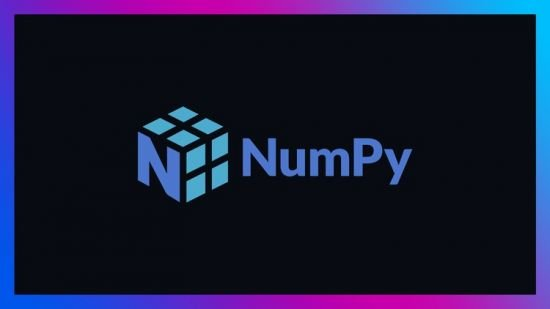﻿ Complete NumPy course with applications 2021 - Python » GFXhome

» » Complete NumPy course with applications 2021 - Python
Information of news
17-06-2021, 04:11

### Complete NumPy course with applications 2021 - Python

Category: TutorialsComplete NumPy course with applications 2021 - Python
MP4 | Video: h264, 1280x720 | Audio: AAC, 44.1 KHz, 2 Ch
Genre: eLearning | Language: English + srt | Duration: 12 lectures (1h 41m) | Size: 523.9 MB

Master Python's central data science and scientific computing library: NumPy
What you'll learn:
How to solve math / statistics problems using NumPy.
Perform the most common array manipulation operations in Machine Learning / Data Science.
Solve problems common to linear algebra, statistics and image processing using the NumPy library.

Requirements
Basic notions of Python programming.

Description
In this course you will learn to use the NumPy library fluently. NumPy is a numerical computation library extensively used in data science, machine learning and statistics. In fact, many other libraries in these fields rely on NumPy arrays to deliver their functionality efficiently. In the area of data science and machine learning we often work with tabular data, which can be represented very well by NumPy arrays. In the course you will learn how to work with n-dimensional arrays and how to manipulate them comfortably to solve complex tasks in different domains.

NumPy processes matrix operations extremely efficiently, offering low execution time and memory usage. Its functionality is implemented in the C programming language: a very efficient compiled language. This functionality is executed from the Python interface with a simple declarative syntax.

The course is divided into 12 lessons:

- Introduction to the NumPy library.

- Creating, indexing and slicing NumPy arrays.

- Copying and editing NumPy arrays.

- Stacking and restructuring NumPy arrays.

- Arithmetic operations with NumPy arrays.

- Operations with NumPy arrays of different shapes.

- Concatenation, reversion and persistence of NumPy arrays.

- Applications of NumPy - Random number generation

- Applications of NumPy - Statistics

- Applications of NumPy - Linear algebra

- Applications of NumPy - Image manipulation

- Applications of NumPy - Chaotic dynamical systems

At the end of the course, you will know how to create arrays using different methods, manipulate them and perform mathematical operations with them.

Who this course is for
Data science students.
Professionals of any scientific or engineering discipline.
Programmers interested in machine learning.
Data analysts interested in expanding their knowledge.

or
https://rapidgator.net/file/dde385518e937a1b8feca4ae701a2564/Complete_NumPy_course_with_applications_2021_-_Python.rar.htmlSite BBcode/HTML Code:
Dear visitor, you went to the site as unregistered user.